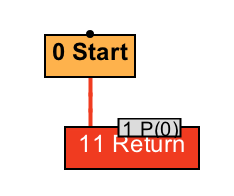24 字段访问相关优化

class Foo {
int a = 0;
}

static int bar(int x) {
Foo foo = new Foo();
foo.a = x;
return foo.a;
}

static int bar(int x) {
int a = x;
return a;
}# {method} 'bar' '(I)I' in 'FieldAccessTest'
# parm0:    rsi       = int             // 参数 x
#           [sp+0x20]  (sp of caller)
0x06a0: sub    rsp,0x18                   // 创建方法栈桢
0x06a7: mov    QWORD PTR [rsp+0x10],rbp   // 无关指令
0x06ac: mov    eax,esi                    // 将参数 x 存入返回值 eax 中
0x06b2: pop    rbp                        // 无关指令
0x06b3: mov    r10,QWORD PTR [r15+0x70]   // 安全点测试
0x06b7: test   DWORD PTR [r10],eax        // 安全点测试
0x06ba: ret

static int bar(Foo o, int x) {
o.a = x;
return o.a;
}

static int bar(Foo o, int x) {
o.a = x;
return x;
}

字段读取优化

static int bar(Foo o, int x) {
int y = o.a + x;
return o.a + y;
}

static int bar(Foo o, int x) {
int t = o.a;
int y = t + x;
return t + y;
}

static int bar(Foo o, int x) {
o.a = 1;
if (o.a >= 0)
return x;
else
return -x;
}

static int bar(Foo o, int x) {
o.a = 1;
return x;
}

class Foo {
boolean a;
void bar() {
a = true;
while (a) {}
}
void whatever() { a = false; }
}

void bar() {
a = true;
while (true) {}
}
// 生成的机器码将陷入这一死循环中
0x066b: mov    r11,QWORD PTR [r15+0x70] // 安全点测试
0x066f: test   DWORD PTR [r11],eax      // 安全点测试
0x0672: jmp    0x066b                   // while (true)

0x00e0: movzx  r11d,BYTE PTR [rbx+0xc]   // 读取 a
0x00e5: mov    r10,QWORD PTR [r15+0x70]  // 安全点测试
0x00e9: test   DWORD PTR [r10],eax       // 安全点测试
0x00ec: test   r11d,r11d                 // while (a)
0x00ef: jne    0x00e0                    // while (a)

class Foo {
int a = 0;
void bar() {
a = 1;
a = 2;
}
}

void bar() {
a = 2;
}

class Foo {
int a = 0;
void bar() {
a = 1;
int t = a;
a = t + 2;
}
}
// 优化为
class Foo {
int a = 0;
void bar() {
a = 1;
int t = 1;
a = t + 2;
}
}
// 进一步优化为
class Foo {
int a = 0;
void bar() {
a = 3;
}
}

死代码消除

int bar(int x, int y) {
int t = x*y;
t = x+y;
return t;
}

int bar(int x, int y) {
return x+y;
}

int bar(boolean f, int x, int y) {
int t = x*y;
if (f)
t = x+y;
return t;
}

int bar(boolean f, int x, int y) {
int t;
if (f)
t = x+y;
else
t = x*y;
return t;
}

int bar(int x) {
if (false)
return x;
else
return -x;
}

int bar(int x) {
return -x;
}

总结与实践

int bar(int x, int y) {
int t = x/y;
t = x+y;
return t;
}